Want to share your content on R-bloggers? click here if you have a blog, or here if you don't.

Time series prediction (forecasting) has experienced dramatic improvements in predictive accuracy as a result of the data science machine learning and deep learning evolution. As these ML/DL tools have evolved, businesses and financial institutions are now able to forecast better by applying these new technologies to solve old problems. In this article, we showcase the use of a special type of Deep Learning model called an LSTM (Long Short-Term Memory), which is useful for problems involving sequences with autocorrelation. We analyze a famous historical data set called “sunspots” (a sunspot is a solar phenomenon wherein a dark spot forms on the surface of the sun). We’ll show you how you can use an LSTM model to predict sunspots ten years into the future with an LSTM model.

## Tutorial Overview

This is an advanced tutorial implementing deep learning for time series and several other complex machine learning topics such as backtesting cross validation. For those seeking an introduction to Keras in R, please check out Customer Analytics: Using Deep Learning With Keras To Predict Customer Churn.

In this tutorial, you will learn how to:

• Develop a Stateful LSTM Model with the `keras` package, which connects to the R TensorFlow backend.
• Apply a Keras Stateful LSTM Model to a famous time series, Sunspots.
• Perform Time Series Cross Validation using Backtesting with the `rsample` package rolling forecast origin resampling.
• Visualize Backtest Sampling Plans and Prediction Results with `ggplot2` and `cowplot`.
• Evaluate whether or not a time series may be a good candidate for an LSTM model by reviewing the Autocorrelation Function (ACF) plot.

The end result is a high performance deep learning algorithm that does an excellent job at predicting ten years of sunspots! Here’s the plot of the Backtested Keras Stateful LSTM Model.Time series prediction (forecasting) has a dramatic effect on the top and bottom line. In business, we could be interested in predicting which day of the month, quarter, or year that large expenditures are going to occur or we could be interested in understanding how the consumer price index (CPI) will change over the course of the next six months. These are common questions that impact organizations both on a microeconomic and macroeconomic level. While the data set used in this tutorial is not a “business” data set, it shows the power of the tool-problem fit, meaning that using the right tool for the job can offer tremendous improvements in accuracy. The net result is increased prediction accuracy ultimately leads to quantifiable improvements to the top and bottom line.

## Long Short-Term Memory (LSTM) Models

A Long Short-Term Memory (LSTM) model is a powerful type of recurrent neural network (RNN). The blog article, “Understanding LSTM Networks”, does an excellent job at explaining the underlying complexity in an easy to understand way. Here’s an image depicting the LSTM internal cell architecture that enables it to persist for long term states (in addition to short term), which traditional RNN’s have difficulty with:Source: Understanding LSTM Networks

LSTMs are quite useful in time series prediction tasks involving autocorrelation, the presence of correlation between the time series and lagged versions of itself, because of their ability to maintain state and recognize patterns over the length of the time series. The recurrent architecture enables the states to persist, or communicate between updates of the weights as each epoch progresses. Further, the LSTM cell architecture enhances the RNN by enabling long term persistence in addition to short term, which is fascinating!

In Keras, LSTM’s can be operated in a “stateful” mode, which according to the Keras documentation:

The last state for each sample at index i in a batch will be used as initial state for the sample of index i in the following batch

In normal (or “stateless”) mode, Keras shuffles the samples, and the dependencies between the time series and the lagged version of itself are lost. However, when run in “stateful” mode, we can often get high accuracy results by leveraging the autocorrelations present in the time series.

We’ll explain more as we go through this tutorial. For now, just understand that LSTM’s can be really useful for time series problems involving autocorrelation and Keras has the capability to create stateful LSTMs that are perfect for time series modeling.

## Sunspots Data Set

Sunspots is a famous data set that ships with R (refer to the `datasets` library). The dataset tracks sunspots, which are the occurrence of a dark spot on the sun. Here’s an image from NASA showing the solar phenomenon. Pretty cool!Source: NASA

The dataset we use in this tutorial is called `sunspots.month`, and it contains 265 years (from 1749 through 2013) of monthly data on number of sunspots per month.## Implementing An LSTM To Predict Sunspots

Time to get to business. Let’s predict sunspots. Here’s our objective:

Objective: Use an LSTM model to generate a forecast of sunspots that spans 10-years into the future.

### 1.0 Libraries

Here are the libraries needed for this tutorial. All are available on CRAN. You can install with `install.packages()` if you do not have them installed already. NOTE: Before proceeding with this code tutorial, make sure to update all packages as previous versions of these packages may not be compatible with the code used. One issue we are aware of is upgrading to `lubridate` version 1.7.4.

If you have not previously run Keras in R, you will need to install Keras using the `install_keras()` function.

### 2.0 Data

The dataset, `sunspot.month`, is available for all of us (it ships with base R). It’s a `ts` class (not tidy), so we’ll convert to a tidy data set using the `tk_tbl()` function from `timetk`. We use this instead of `as.tibble()` from `tibble` to automatically preserve the time series index as a `zoo` `yearmon` index. Last, we’ll convert the `zoo` index to date using `lubridate::as_date()` (loaded with `tidyquant`) and then change to a `tbl_time` object to make time series operations easier.

### 3.0 Exploratory Data Analysis

The time series is long (265 years!). We can visualize the time series both full (265 years) and zoomed in on the first 50 years to get a feel for the series.

#### 3.1 Visualizing Sunspot Data With Cowplot

We’ll make to `ggplot`s and combine them using `cowplot::plot_grid()`. Note that for the zoomed in plot, we make use of `tibbletime::time_filter()`, which is an easy way to perform time-based filtering.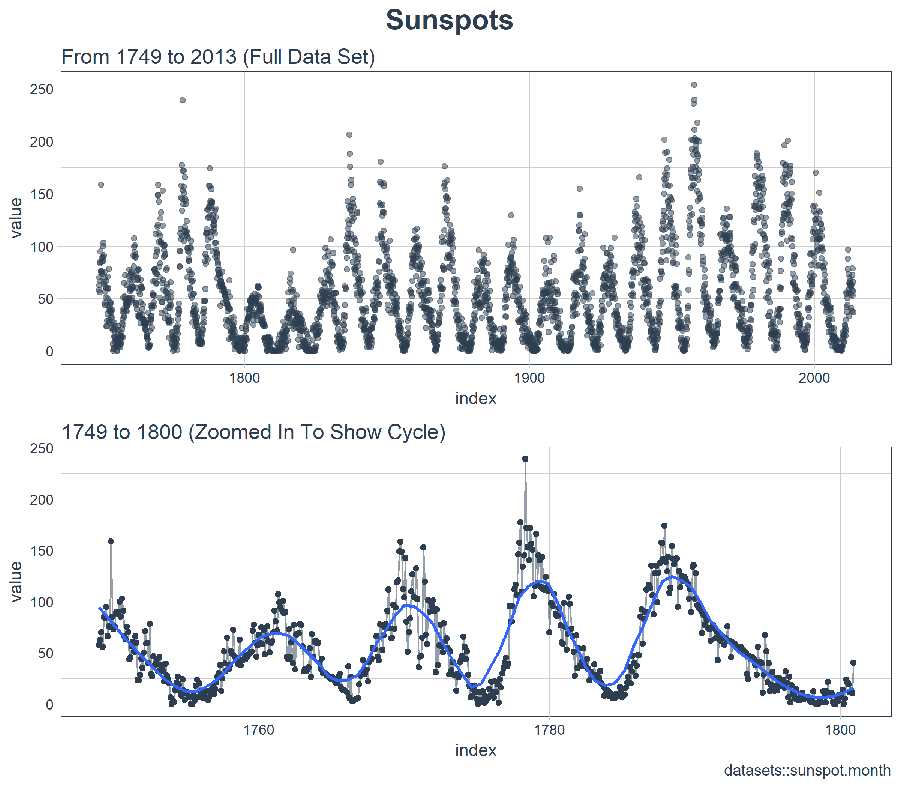At first glance, it looks like this series should be easy to predict. However, we can see that the cycle (10-year frequency) and amplitude (count of sunspots) seems to change at least between years 1780 and 1800. This creates some challenges.

#### 3.2 Evaluating The ACF

The next thing we can do is determine whether or not an LSTM model may be a good approach. The LSTM will leverage autocorrelation to generate sequence predictions. Our goal is to produce a 10-year forecast using batch forecasting (a technique for creating a single forecast batch across the forecast region, which is in contrast to a single-prediction that is iteratively performed one or several steps into the future). The batch prediction will only work if the autocorrelation used is beyond ten years. Let’s inspect.

First, we need to review the Autocorrelation Function (ACF), which is the correlation between the time series of interest in lagged versions of itself. The `acf()` function from the `stats` library returns the ACF values for each lag as a plot. However, we’d like to get the ACF values as data so we can investigate the underlying data. To do so, we’ll create a custom function, `tidy_acf()`, to return the ACF values in a tidy tibble.

Next, let’s test the function out to make sure it works as intended. The function takes our tidy time series, extracts the value column, and returns the ACF values along with the associated lag in a tibble format. We have 601 autocorrelation (one for the time series and it’s 600 lags). All looks good.

Let’s plot the ACF with `ggplot2` to determine if a high-autocorrelation lag exists beyond 10 years.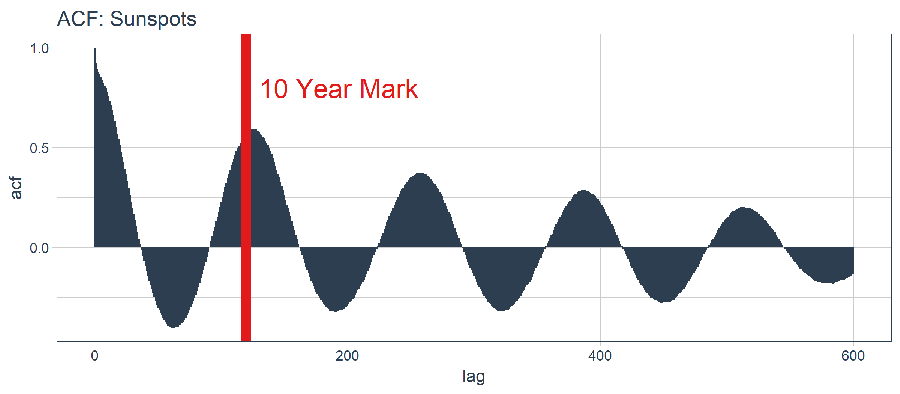This is good news. We have autocorrelation in excess of 0.5 beyond lag 120 (the 10-year mark). We can theoretically use one of the high autocorrelation lags to develop an LSTM model.Upon inspection, the optimal lag occurs at lag 125. This isn’t necessarily the one we will use since we have more to consider with batch forecasting with a Keras LSTM. With that said, here’s how you can `filter()` to get the best lag.

### 4.0 Backtesting: Time Series Cross Validation

Cross validation is the process of developing models on sub-sampled data against a validation set with the goal of determining an expected accuracy level and error range. Time series is a bit different than non-sequential data when it comes to cross validation. Specifically, the time dependency on previous time samples must be preserved when developing a sampling plan. We can create a cross validation sampling plan using by offsetting the window used to select sequential sub-samples. In finance, this type of analysis is often called “Backtesting”, which takes a time series and splits it into multiple uninterupted sequences offset at various windows that can be tested for strategies on both current and past observations.

A recent development is the `rsample` package, which makes cross validation sampling plans very easy to implement. Further, the `rsample` package has Backtesting covered. The vignette, “Time Series Analysis Example”, describes a procedure that uses the `rolling_origin()` function to create samples designed for time series cross validation. We’ll use this approach.

#### 4.1 Developing A Backtesting Strategy

The sampling plan we create uses 50 years (`initial` = 12 x 50 samples) for the training set and ten years (`assess` = 12 x 10) for the testing (validation) set. We select a `skip` span of twenty years (`skip` = 12 x 20) to evenly distribute the samples into 11 sets that span the entire 265 years of sunspots history. Last, we select `cumulative = FALSE` to allow the origin to shift which ensures that models on more recent data are not given an unfair advantage (more observations) that those on less recent data. The tibble return contains the `rolling_origin_resamples`.

#### 4.2 Visualizing The Backtesting Strategy

We can visualize the resamples with two custom functions. The first, `plot_split()`, plots one of the resampling splits using `ggplot2`. Note that an `expand_y_axis` argument is added to expand the date range to the full `sun_spots` dataset date range. This will become useful when we visualize all plots together.

The `plot_split()` function takes one split (in this case Slice01), and returns a visual of the sampling strategy. We expand the axis to the range for the full dataset using `expand_y_axis = TRUE`.The second function, `plot_sampling_plan()`, scales the `plot_split()` function to all of the samples using `purrr` and `cowplot`.

We can now visualize the ENTIRE BACKTESTING STRATEGY with `plot_sampling_plan()`! We can see how the sampling plan shifts the sampling window with each progressive slice of the train/test splits.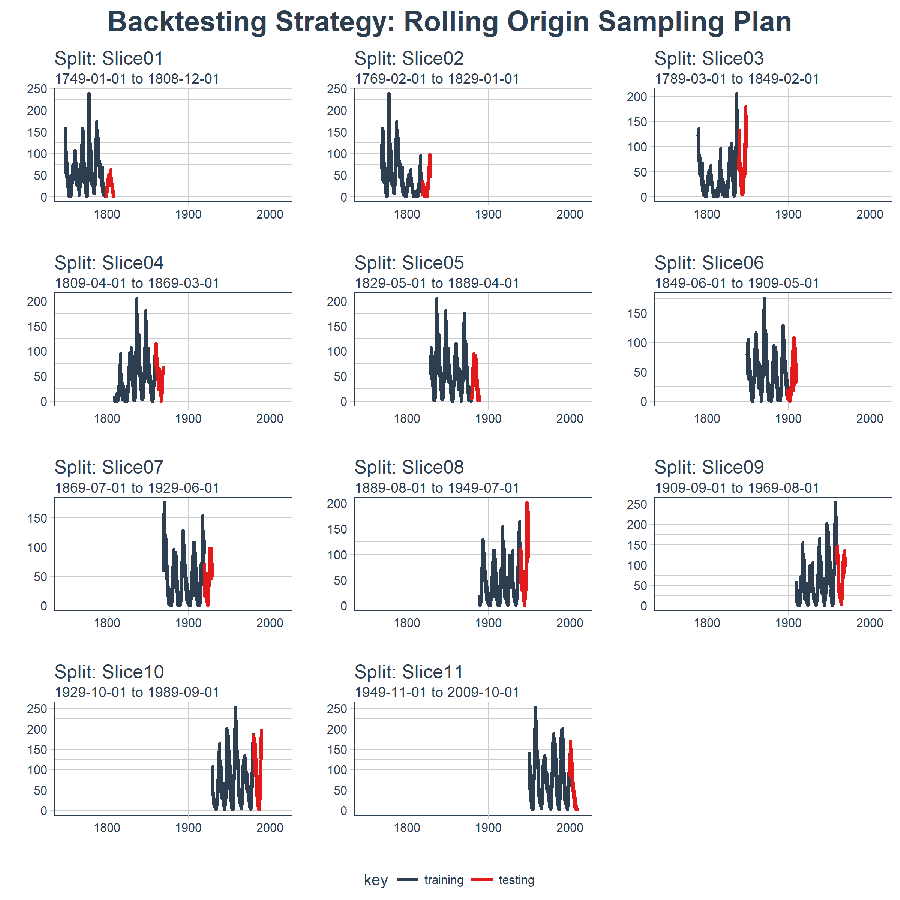And, we can set `expand_y_axis = FALSE` to zoom in on the samples.We’ll use this Backtesting Strategy (11 samples from one time series each with 50/10 split in years and a 20 year offset) when testing the veracity of the LSTM model on the sunspots dataset.

### 5.0 Modeling The Keras Stateful LSTM Model

To begin, we’ll develop a single Keras Stateful LSTM model on a single sample from the Backtesting Strategy. We’ll then scale the model to all samples to investigate/validate the modeling performance.

#### 5.1 Single LSTM

For the single LSTM model, we’ll select and visualize the split for the most recent time sample/slice (Slice11). The 11th split contains the most recent data.

##### 5.1.1 Visualizing The Split

We can reuse the `plot_split()` function to visualize the split. Set `expand_y_axis = FALSE` to zoom in on the subsample.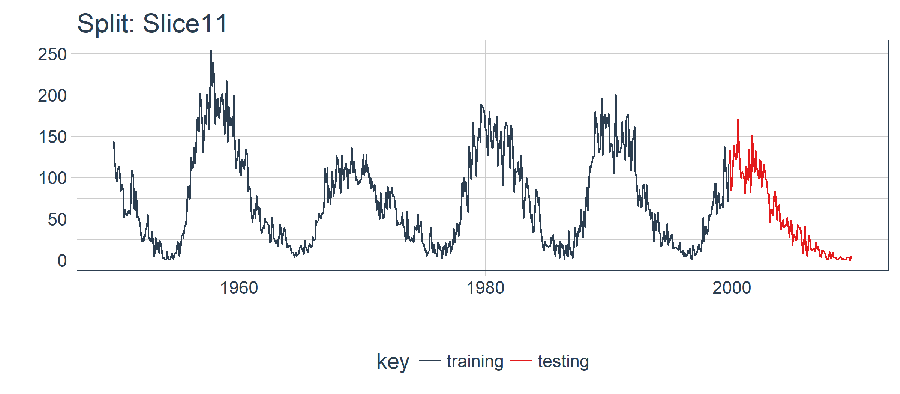##### 5.1.2 Data Setup

First, let’s combine the training and testing data sets into a single data set with a column `key` that specifies what set they came from (either “training” or “testing)”. Note that the `tbl_time` object will need to have the index re-specified during the `bind_rows()` step, but this issue should be corrected in `dplyr` soon (please upvote it so RStudio focuses on it).

##### 5.1.3 Preprocessing With Recipes

The LSTM algorithm requires the input data to be centered and scaled. We can preprocess the data using the `recipes` package. We’ll use a combination of `step_sqrt` to transform the data and reduce the presence of outliers and `step_center` and `step_scale` to center and scale the data. The data is processed/transformed using the `bake()` function.

Next, let’s capture the center/scale history so we can invert the center and scaling after modeling. The square-root transformation can be inverted by squaring the inverted center/scale values.

##### 5.1.4 LSTM Plan

We need a plan for building the LSTM. First, a couple PRO TIPS with LSTM’s:

Tensor Format:

• Predictors (X) must be a 3D Array with dimensions: [samples, timesteps, features]: The first dimension is the length of values, the second is the number of time steps (lags), and the third is the number of predictors (1 if univariate or n if multivariate)
• Outcomes/Targets (y) must be a 2D Array with dimensions: [samples, timesteps]: The first dimension is the length of values and the second is the number of time steps (lags)

Training/Testing:

• The training and testing length must be evenly divisible (e.g. training length / testing length must be a whole number)

Batch Size:

• The batch size is the number of training examples in one forward/backward pass of a RNN before a weight update
• The batch size must be evenly divisible into both the training an testing lengths (e.g. training length / batch size and testing length / batch size must both be whole numbers)

Time Steps:

• A time step is the number of lags included in the training/testing set
• For our example, our we use a single lag

Epochs:

• The epochs are the total number of forward/backward pass iterations
• Typically more improves model performance unless overfitting occurs at which time the validation accuracy/loss will not improve

Taking this in, we can come up with a plan. We’ll select a prediction of window 120 months (10 years) or the length of our test set. The best correlation occurs at 125, but this is not evenly divisible by the forecasting range. We could increase the forecast horizon, but this offers a minimal increase in autocorrelation. We can select a batch size of 40 units which evenly divides into the number of testing and training observations. We select time steps = 1, which is because we are only using one lag. Finally, we set `epochs = 300`, but this will need to be adjusted to balance the bias/variance tradeoff.

##### 5.1.5 2D And 3D Train/Test Arrays

We can then setup the training and testing sets in the correct format (arrays) as follows. Remember, LSTM’s need 3D arrays for predictors (X) and 2D arrays for outcomes/targets (y).

##### 5.1.6 Building The LSTM Model

We can build an LSTM model using the `keras_model_sequential()` and adding layers like stacking bricks. We’ll use two LSTM layers each with 50 units. The first LSTM layer takes the required input shape, which is the [time steps, number of features]. The batch size is just our batch size. We set the first layer to `return_sequences = TRUE` and `stateful = TRUE`. The second layer is the same with the exception of `batch_size`, which only needs to be specified in the first layer, and `return_sequences = FALSE` which does not return the time stamp dimension (2D shape is returned versus a 3D shape from the first LSTM). We tack on a `layer_dense(units = 1)`, which is the standard ending to a keras sequential model. Last, we `compile()` using the `loss = "mae"` and the popular `optimizer = "adam"`.

##### 5.1.7 Fitting The LSTM Model

Next, we can fit our stateful LSTM using a `for` loop (we do this to manually reset states). This will take a minute or so for 300 epochs to run. We set `shuffle = FALSE` to preserve sequences, and we manually reset the states after each epoch using `reset_states()`.

##### 5.1.8 Predicting Using The LSTM Model

We can then make predictions on the test set, `x_test_arr`, using the `predict()` function. We can retransform our predictions using the `scale_history` and `center_history`, which were previously saved and then squaring the result. Finally, we combine the predictions with the original data in one column using `reduce()` and a custom `time_bind_rows()` function.

##### 5.1.9 Assessing Performance Of The LSTM On A Single Split

We can use the `yardstick` package to assess performance using the `rmse()` function, which returns the root mean squared error (RMSE). Our data is in the long format (optimal format for visualizing with `ggplot2`), so we’ll create a wrapper function `calc_rmse()` that processes the data into the format needed for `yardstick::rmse()`.

We can inspect the RMSE on the model.

The RMSE doesn’t tell us the story. We need to visualize. Note - The RMSE will come in handy in determining an expected error when we scale to all samples in the backtesting strategy.

##### 5.1.10 Visualizing The Single Prediction

Next, we make a plotting function, `plot_prediction()`, using `ggplot2` to visualize the results for a single sample.

We can test out the plotting function setting the `id = split_id`, which is “Slice11”.The LSTM performed relatively well! The settings we selected seem to produce a decent model that picked up the trend. It jumped the gun on the next up-trend, but overall it exceeded my expectations. Now, we need to Backtest to see the true performance over time!

#### 5.2 Backtesting The LSTM On All Eleven Samples

Once we have the LSTM working for one sample, scaling to all 11 is relatively simple. We just need to create an prediction function that can be mapped to the sampling plan data contained in `rolling_origin_resamples`.

##### 5.2.1 Creating An LSTM Prediction Function

This step looks intimidating, but it’s actually straightforward. We copy the code from Section 5.1 into a function. We’ll make it a safe function, which is a good practice for any long-running mapping processes to prevent a single failure from stopping the entire process.

We can test the custom `predict_keras_lstm()` function out with 10 epochs. It returns the data in long format with “actual” and “predict” values in the key column.

##### 5.2.2 Mapping The LSTM Prediction Function Over The 11 Samples

With the `predict_keras_lstm()` function in hand that works on one split, we can now map to all samples using a `mutate()` and `map()` combo. The predictions will be stored in a “list” column called “predict”. Note that this will take 5-10 minutes or so to complete.

We now have the predictions in the column “predict” for all 11 splits!.

##### 5.2.3 Assessing The Backtested Performance

We can assess the RMSE by mapping the `calc_rmse()` function to the “predict” column.And, we can summarize the RMSE for the 11 slices. PRO TIP: Using the average and standard deviation of the RMSE (or other similar metric) is a good way to compare the performance of various models.

##### 5.2.4 Visualizing The Backtest Results

We can create a `plot_predictions()` function that returns one plot with the predictions for the entire set of 11 backtesting samples!!!

Here’s the result. On a data set that’s not easy to predict, this is quite impressive!!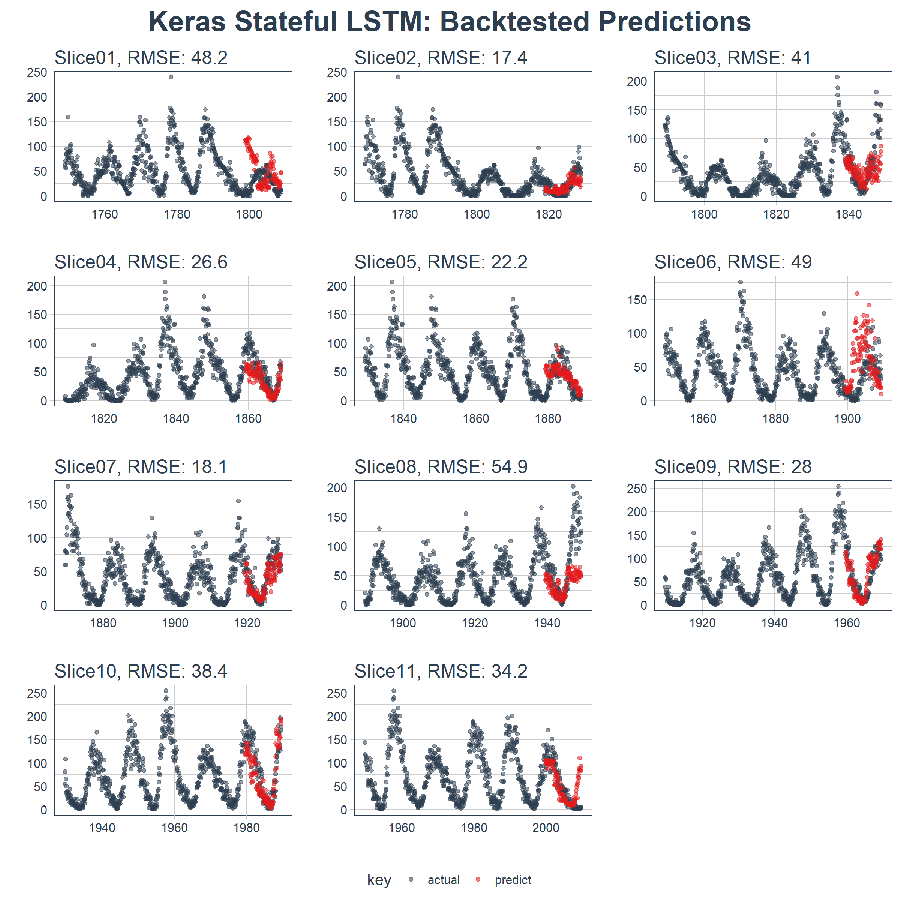#### 5.3 Predicting The Next 10 Years

We can predict the next 10 years by adjusting the prediction function to work with the full data set. A new function, `predict_keras_lstm_future()`, is created that predicts the next 120 time steps (or ten years).

Next, run `predict_keras_lstm_future()` on the `sun_spots` data set.

Last, we can visualize the forecast with the `plot_prediction()` function, setting `id = NULL`. We can use `filter_time()` to zoom in on the dataset since 1900.## Conclusions

This article demonstrates the power of a Stateful LSTM built using the `keras` package. It’s surprising how well the deep learning approach learned the trend given that the only feature provided was the lag at 120. The backtested model had an Average RMSE of 34 and a Standard Deviation RMSE of 13. While not shown in the article, we tested this against ARIMA and prophet, and the LSTM outperformed both by a more than 30% reduction in average error and 40% reduction in standard deviation. This shows the benefit of machine learning tool-application fit.

Beyond the deep learning approach used, the article also exposed methods to determine whether an LSTM is a good option for the given time series using ACF plot. We also exposed how the veracity of a time series model should be benchmarked by backtesting, a strategy that can be used for cross validation that preserves the sequential aspects of the time series.

We are nearing the roll out of our Data Science For Business Virtual Workshop that teaches data scientists how we implement data science for business (DS4B). We walk the student through a real-world problem: Employee Attrition (turnover), implementing various machine learning techniques including `h2o` and `lime` along with a full course on `Shiny` web app development of an Employee Attrition Smart Scorecard.

Don’t wait. Enroll in Business Science University today! You’ll get an early-bird discount on the first Virtual Workshop.

### DS4B Virtual Workshop: Predicting Employee Attrition

Did you know that an organization that loses 200 high performing employees per year is essentially losing \$15M/year in lost productivity? Many organizations don’t realize this because it’s an indirect cost. It goes unnoticed. What if you could use data science to predict and explain turnover in a way that managers could make better decisions and executives would see results? You will learn the tools to do so in our Virtual Workshop. Here’s an example of a Shiny app you will create.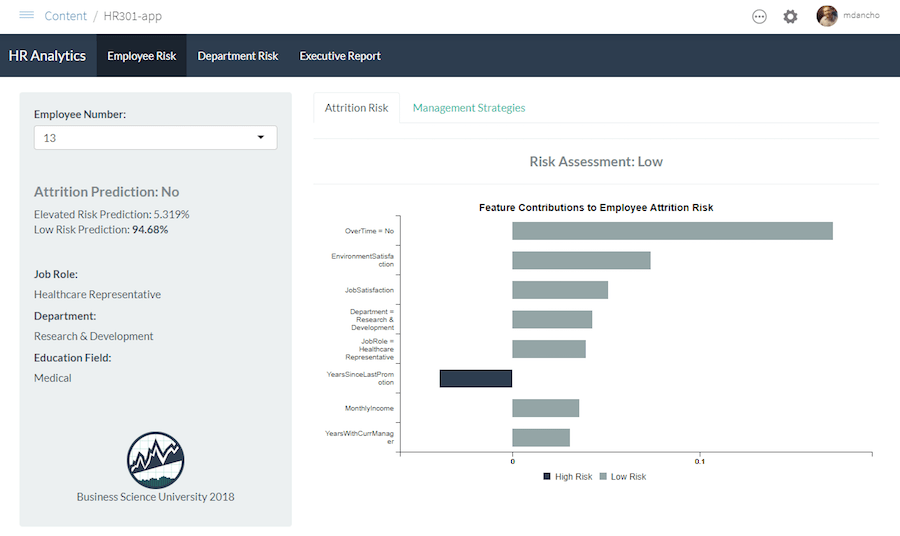Shiny App That Predicts Attrition and Recommends Management Strategies, Taught in HR 301

Our first Data Science For Business Virtual Workshop teaches you how to solve this employee attrition problem in four courses that are fully integrated:

• HR 201: Predicting Employee Attrition with `h2o` and `lime`
• HR 301: Building A `Shiny` Web Application
• HR 302: Data Story Telling With `RMarkdown` Reports and Presentations
• HR 303: Building An R Package For Your Organization, `tidyattrition`

The Virtual Workshop is intended for intermediate and advanced R users. It’s code intensive (like these articles), but also teaches you fundamentals of data science consulting including CRISP-DM and the Business Science Problem Framework. The content bridges the gap between data science and the business, making you even more effective and improving your organization in the process.

Interested? Enroll in Business Science University today!

## New R Package: Anomalize For Scalable Time Series Anomaly Detection

We just released our package, `anomalize`. You can learn more from the Anomalize Documentation or by watching our 2-minute introductory video!

## Don’t Miss A Beat

If you like our software (`anomalize`, `tidyquant`, `tibbletime`, `timetk`, and `sweep`), our courses, and our company, you can connect with us: